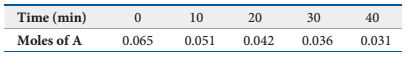### Create an Account

Home / Questions / Consider the following hypothetical aqueous reaction: A(aq) B(aq). A flask is charged wit...

# Consider the following hypothetical aqueous reaction: A(aq) B(aq). A flask is charged with 0.065 mol of A in a total volume of 100.0 mL. The following data are collected: (a) Calculate the number

Consider the following hypothetical aqueous reaction: A(aqB(aq). A flask is charged with 0.065 mol of A in a total volume of 100.0 mL. The following data are collected:(a) Calculate the number of moles of B at each time in the table, assuming that there are no molecules of B at time zero, and that A cleanly converts to B with no intermediates. (b) Calculate the average rate of disappearance of A for each 10-min interval in units of M/s. (c) Between t = 10 min and t = 30 min, what is the average rate of appearance of B in units of M/s? Assume that the volume of the solution is constant.

Aug 21 2021 View more View LessSubscribe To Get Solution